5G时代下一个聚合的编程学习网

# The 7 most popular ways to plot data in Python

This guide will help you decide. It will show you how to use each of the four most popular Python plotting libraries—Matplotlib, Seaborn, Plotly, and Bokeh—plus a couple of great up-and-comers to consider: Altair, with its expressive API, and Pygal, with its beautiful SVG output. I’ll also look at the very convenient plotting API provided by pandas.

For each library, I’ve included source code snippets, as well as a full web-based example using Anvil, our platform for building web apps with nothing but Python. Let’s take a look.

## An example plot

Each library takes a slightly different approach to plotting data. To compare them, I’ll make the same plot with each library and show you the source code. For my example data, I chose this grouped bar chart of British election results since 1966:

## british-election-data-chart.png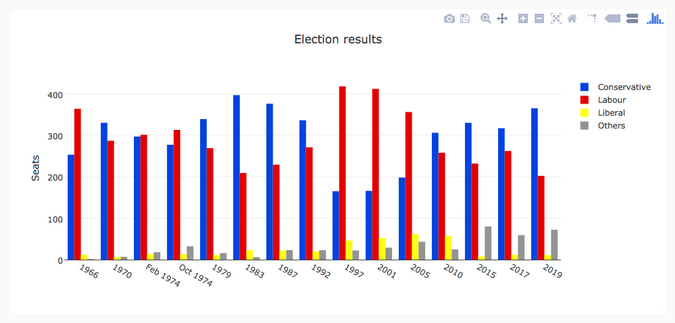I compiled the dataset of British election history from Wikipedia: the number of seats in the UK parliament won by the Conservative, Labour, and Liberal parties (broadly defined) in each election from 1966 to 2019, plus the number of seats won by “others.” You can download it as a CSV file.

## Matplotlib

Matplotlib is the oldest Python plotting library, and it’s still the most popular. It was created in 2003 as part of the SciPy Stack, an open source scientific computing library similar to Matlab.

Matplotlib gives you precise control over your plots—for example, you can define the individual x-position of each bar in your barplot. Here is the code to graph this (which you can run here):

import matplotlib.pyplot as plt
import numpy as np
from votes import wide as df

# Initialise a figure. subplots() with no args gives one plot.
fig, ax = plt.subplots()

# A little data preparation
years = df[‘year’]
x = np.arange(len(years))

# Plot each bar plot. Note: manually calculating the ‘dodges’ of the bars
ax.bar(x – 3*width/2, df[‘conservative’], width, label=‘Conservative’, color=‘#0343df’)
ax.bar(x – width/2, df[‘labour’], width, label=‘Labour’, color=‘#e50000’)
ax.bar(x + width/2, df[‘liberal’], width, label=‘Liberal’, color=‘#ffff14’)
ax.bar(x + 3*width/2, df[‘others’], width, label=‘Others’, color=‘#929591’)

# Customise some display properties
ax.set_ylabel(‘Seats’)
ax.set_title(‘UK election results’)
ax.set_xticks(x)    # This ensures we have one tick per year, otherwise we get fewer
ax.set_xticklabels(years.astype(str).values, rotation=‘vertical’)
ax.legend()

# Ask Matplotlib to show the plot
plt.show()

And here are the election results plotted in Matplotlib:

## matplotlib.png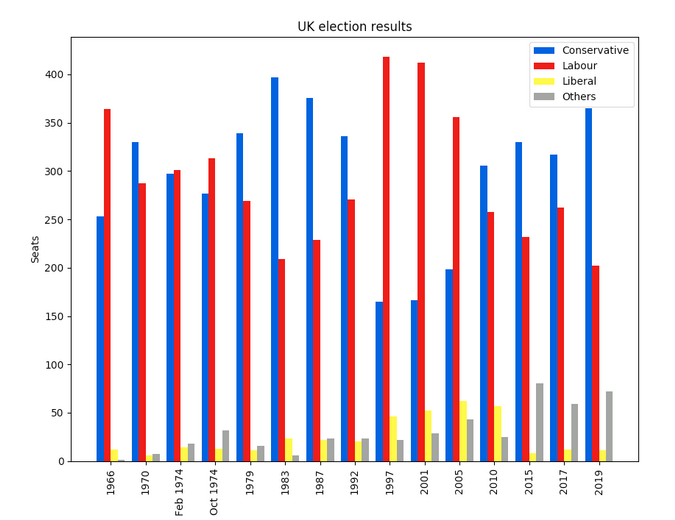## Seaborn

Seaborn is an abstraction layer on top of Matplotlib; it gives you a really neat interface to make a wide range of useful plot types very easily.

It doesn’t compromise on power, though! Seaborn gives escape hatches to access the underlying Matplotlib objects, so you still have complete control.

Seaborn’s code is simpler than the raw Matplotlib (runnable here):

import seaborn as sns
from votes import long as df

# Some boilerplate to initialise things
sns.set()
plt.figure()

# This is where the actual plot gets made
ax = sns.barplot(data=df, x=“year”, y=“seats”, hue=“party”, palette=[‘blue’, ‘red’, ‘yellow’, ‘grey’], saturation=0.6)

# Customise some display properties
ax.set_title(‘UK election results’)
ax.grid(color=‘#cccccc’)
ax.set_ylabel(‘Seats’)
ax.set_xlabel(None)
ax.set_xticklabels(df[“year”].unique().astype(str), rotation=‘vertical’)

# Ask Matplotlib to show it
plt.show()

And produces this chart:

## seaborn.png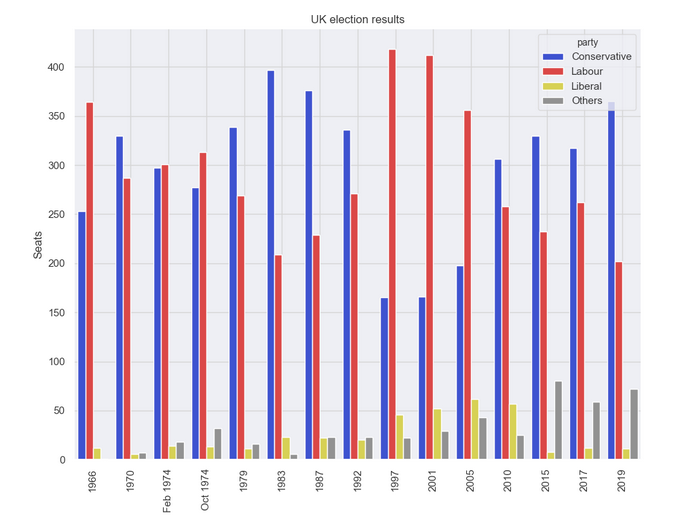## Plotly

Plotly is a plotting ecosystem that includes a Python plotting library. It has three different interfaces:

• An object-oriented interface
• An imperative interface that allows you to specify your plot using JSON-like data structures
• A high-level interface similar to Seaborn called Plotly Express

Plotly plots are designed to be embedded in web apps. At its core, Plotly is actually a JavaScript library! It uses D3 and stack.gl to draw the plots.

You can build Plotly libraries in other languages by passing JSON to the JavaScript library. The official Python and R libraries do just that. At Anvil, we ported the Python Plotly API to run in the web browser.

Here’s the source code in Plotly (which you can run here):

import plotly.graph_objects as go
from votes import wide as df

#  Get a convenient list of x-values
years = df[‘year’]
x = list(range(len(years)))

# Specify the plots
bar_plots = [
go.Bar(x=x, y=df[‘conservative’], name=‘Conservative’, marker=go.bar.Marker(color=‘#0343df’)),
go.Bar(x=x, y=df[‘labour’], name=‘Labour’, marker=go.bar.Marker(color=‘#e50000’)),
go.Bar(x=x, y=df[‘liberal’], name=‘Liberal’, marker=go.bar.Marker(color=‘#ffff14’)),
go.Bar(x=x, y=df[‘others’], name=‘Others’, marker=go.bar.Marker(color=‘#929591’)),
]

# Customise some display properties
layout = go.Layout(
title=go.layout.Title(text=“Election results”, x=0.5),
yaxis_title=“Seats”,
xaxis_tickmode=“array”,
xaxis_tickvals=list(range(27)),
xaxis_ticktext=tuple(df[‘year’].values),
)

# Make the multi-bar plot
fig = go.Figure(data=bar_plots, layout=layout)

# Tell Plotly to render it
fig.show()

And the election results plot:

## plotly.gif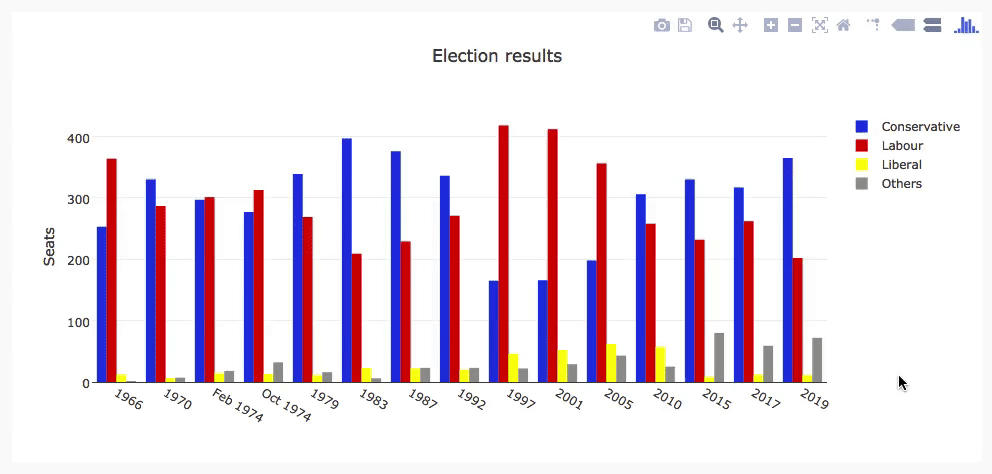## Bokeh

Bokeh (pronounced “BOE-kay”) specializes in building interactive plots, so this standard example doesn’t show it off to its best. Like Plotly, Bokeh’s plots are designed to be embedded in web apps; it outputs its plots as HTML files.

Here is the code in Bokeh (which you can run here)

from bokeh.io import show, output_file
from bokeh.models import ColumnDataSource, FactorRange, HoverTool
from bokeh.plotting import figure
from bokeh.transform import factor_cmap
from votes import long as df

# Specify a file to write the plot to
output_file(“elections.html”)

# Tuples of groups (year, party)
x = [(str(r[‘year’]), r[‘party’]) for r in df.iterrows()]
y = df[‘seats’]

# Bokeh wraps your data in its own objects to support interactivity
source = ColumnDataSource(data=dict(x=x, y=y))

# Create a colourmap
cmap = {
‘Conservative’: ‘#0343df’,
‘Labour’: ‘#e50000’,
‘Liberal’: ‘#ffff14’,
‘Others’: ‘#929591’,
}
fill_color = factor_cmap(‘x’, palette=list(cmap.values()), factors=list(cmap.keys()), start=1, end=2)

# Make the plot
p = figure(x_range=FactorRange(*x), width=1200, title=“Election results”)
p.vbar(x=‘x’, top=‘y’, width=0.9, source=source, fill_color=fill_color, line_color=fill_color)

# Customise some display properties
p.y_range.start = 0
p.yaxis.axis_label = ‘Seats’
p.xaxis.major_label_orientation = 1
p.xgrid.grid_line_color = None

And the plot:

## bokeh.png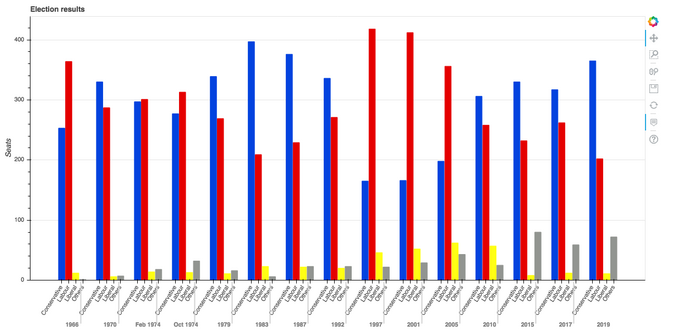## Altair

Altair is based on a declarative plotting language (or “visualization grammar”) called Vega. This means it’s a well-thought-through API that scales well for complex plots, saving you from getting lost in nested-for-loop hell.

As with Bokeh, Altair outputs its plots as HTML files. Here’s the code (which you can run here):

import altair as alt
from votes import long as df

# Set up the colourmap
cmap = {
‘Conservative’: ‘#0343df’,
‘Labour’: ‘#e50000’,
‘Liberal’: ‘#ffff14’,
‘Others’: ‘#929591’,
}

# Cast years to strings
df[‘year’] = df[‘year’].astype(str)

# Here’s where we make the plot
chart = alt.Chart(df).mark_bar().encode(
x=alt.X(‘party’, title=None),
y=‘seats’,
column=alt.Column(‘year’, sort=list(df[‘year’]), title=None),
color=alt.Color(‘party’, scale=alt.Scale(domain=list(cmap.keys()), range=list(cmap.values())))
)

# Save it as an HTML file.
chart.save(‘altair-elections.html’)

And the resulting chart:

## altair.png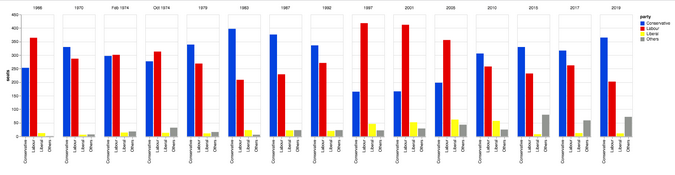## Pygal

Pygal focuses on visual appearance. It produces SVG plots by default, so you can zoom them forever or print them out without them getting pixellated. Pygal plots also come with some good interactivity features built-in, making Pygal another underrated candidate if you’re looking to embed plots in a web app.

The source code looks like this (and you can run it here):

import pygal
from pygal.style import Style
from votes import wide as df

# Define the style
custom_style = Style(
colors=(‘#0343df’, ‘#e50000’, ‘#ffff14’, ‘#929591’)
font_family=‘Roboto,Helvetica,Arial,sans-serif’,
background=‘transparent’,
label_font_size=14,
)

# Set up the bar plot, ready for data
c = pygal.Bar(
title=“UK Election Results”,
style=custom_style,
y_title=‘Seats’,
width=1200,
x_label_rotation=270,
)

# Add four data sets to the bar plot

# Define the X-labels
c.x_labels = df[‘year’]

# Write this to an SVG file
c.render_to_file(‘pygal.svg’)

And the chart:

## pygal.png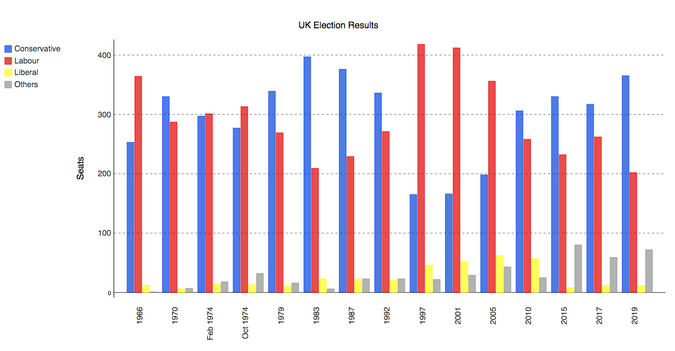## Pandas

Pandas is an extremely popular data science library for Python. It allows you to do all sorts of data manipulation scalably, but it also has a convenient plotting API. Because it operates directly on data frames, the pandas example is the most concise code snippet in this article—even shorter than the Seaborn code!

The pandas API is a wrapper around Matplotlib, so you can also use the underlying Matplotlib API to get fine-grained control of your plots.

Here’s the election results plot in pandas. The code is beautifully concise!

from matplotlib.colors import ListedColormap
from votes import wide as df

cmap = ListedColormap([‘#0343df’, ‘#e50000’, ‘#ffff14’, ‘#929591’])

ax = df.plot.bar(x=‘year’, colormap=cmap)

ax.set_xlabel(None)
ax.set_ylabel(‘Seats’)
ax.set_title(‘UK election results’)

plt.show()

And the resulting chart:

## pandas.png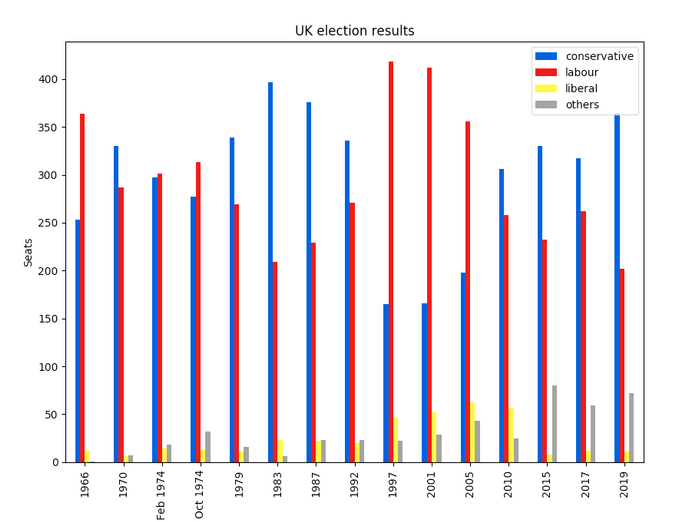To run this example, check out here.

## Plot your way

Python offers many ways to plot the same data without much code. While you can get started quickly creating charts with any of these methods, they do take some local configuration. Anvil offers a beautiful web-based experience for Python development if you’re in need. Happy plotting!

This article is based on Plotting in Python: comparing the options on Anvil’s blog and is reused with permission.

https://opensource.com/article/20/4/plot-data-python